# 555 Astable

The 555 timer IC can be used with a few simple components to build an astable circuit which produces a 'square wave'. This is a digital waveform with sharp transitions between low (0V) and high (+Vs), the durations of the low and high states may be different. The circuit is called an astable because it is not stable in any state: the output is continually changing between 'low' and 'high'.

Recommended book: IC 555 Projects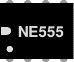Rapid Electronics: NE555 timer (standard)

Rapid Electronics: ICM7555 (low power)

## Time period and frequency

The time period (T) of the square wave is the time for one complete cycle, but it is often better to consider frequency (f) which is the number of cycles per second.

 T = 0.7 × (R1 + 2R2) × C1
 f = 1.4 (R1 + 2R2) × C1

T   = time period in seconds (s)
f    = frequency in hertz (Hz)
R1 = resistance in ohms (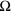)
R2 = resistance in ohms ()
C1 = capacitance in farads (F)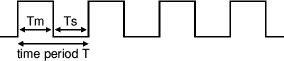555 astable output, a square wave
(Tm and Ts may be different)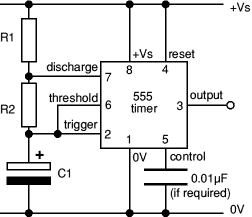555 astable circuit

## Mark and Space times

The time period can be split into two parts:

 Time period, T = Tm + Ts
 Mark time (output high)Tm = 0.7 × (R1 + R2) × C1
 Space time (output low)Ts = 0.7 × R2 × C1

It is important to note that Tm must be greater than Ts since R1 cannot be 0(the minimum is 1k).

Many circuits require Tm and Ts to be about equal. This is achieved if R2 is much larger than R1.

For a standard astable circuit note that Tm will always be greater than Ts because Ts is propotional to R2 but Tm is propotional to R2 + R1 and R1 cannot be zero.

This is not too restricting because the output can both sink and source current. For example an LED can be made to flash briefly with long gaps by connecting it (with its resistor) between +Vs and the output. This way the LED is on during Ts, so brief flashes are achieved with R1 larger than R2, making Ts short and Tm long.

If R1 is much less than R2, Tm and Ts will be very similar and that's usually good enough for circuits which need Tm and Ts to be (roughly) equal.

If Tm must be less than Ts a diode can be added to the circuit as explained under duty cycle below.

### Choosing R1, R2 and C1

R1 and R2 should be in the range 1kto 1M. It is best to choose C1 first because capacitors are available in just a few values.

Choose C1 to suit the frequency range you require (use the table as a guide).

Choose R2 to give the frequency (f) you require. Assume that R1 is much smaller than R2 (so that Tm and Ts are almost equal), then you can use:

 If R1 << R2 use R2 = 0.7 f × C1

Choose R1 to be about a tenth of R2 (the minimum is 1k) unless you want the mark time Tm to be significantly longer than the space time Ts.

If you need a variable resistor it is best to make it R2.

Beware that if R1 is variable it must have a fixed resistor of at least 1kin series (this is not required for R2).

 555 astable frequencies C1 R2 10kR1 1kR2 100kR1 10kR2 1MR1 100k0.001µF 68kHz 6.8kHz 680Hz 0.01µF 6.8kHz 680Hz 68Hz 0.1µF 680Hz 68Hz 6.8Hz 1µF 68Hz 6.8Hz 0.68Hz 10µF 6.8Hz 0.68Hz(41 per min.) 0.068Hz(4 per min.)

## Duty cycle

The duty cycle of an astable circuit is the proportion of the complete cycle for which the output is high (the mark time). It is usually given as a percentage.

For a standard 555 astable circuit the mark time (Tm) must be greater than the space time (Ts), so the duty cycle must be at least 50%:

 Duty cycle  = Tm = R1 + R2 Tm + Ts R1 + 2R2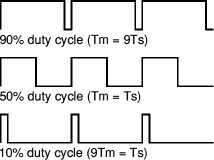### Duty cycle of less than 50%

To achieve a duty cycle of less than 50% a signal diode (such as 1N4148) can be added in parallel with R2 as shown in the diagram. This bypasses R2 during the charging (mark) part of the cycle so that Tm depends only on R1 and C1:

 555 astable with diode (for duty cycle < 50%) Tm = 0.7 × R1 × C1   (ignoring 0.7V across diode) Ts  = 0.7 × R2 × C1   (unchanged) T = Tm + Ts = 0.7 × (R1 + R2) × C1
 Duty cyclewith diode = Tm = R1 Tm + Ts R1 + R2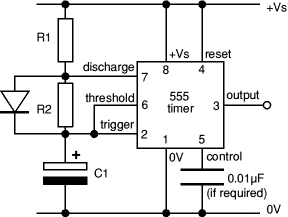555 astable circuit with diode across R2
Tm can be less than Ts so the
duty cycle can be less than 50%

## Astable operation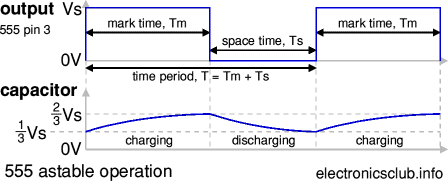With the output high (+Vs) the capacitor C1 is charged by current flowing through R1 and R2.

The threshold and trigger inputs monitor the capacitor voltage and when it reaches 2/3Vs (threshold voltage) the output becomes low and the discharge pin is connected to 0V.

The capacitor now discharges with current flowing through R2 into the discharge pin. When the voltage falls to 1/3Vs (trigger voltage) the output becomes high again and the discharge pin is disconnected, allowing the capacitor to start charging again.

This cycle repeats continuously unless the reset input is connected to 0V which forces the output low while reset is 0V.

An astable can be used to provide the clock signal for circuits such as counters.

A low frequency astable (< 10Hz) can be used to flash an LED on and off, higher frequency flashes are too fast to be seen clearly. Driving a loudspeaker or piezo transducer with a low frequency of less than 20Hz will produce a series of 'clicks' (one for each low/high transition) and this can be used to make a simple metronome.

An audio frequency astable (20Hz to 20kHz) can be used to produce a sound from a loudspeaker or piezo transducer. The sound is suitable for buzzes and beeps. The natural (resonant) frequency of most piezo transducers is about 3kHz and this will make them produce a particularly loud sound.

#### Projects using 555 astable:

Rapid Electronics: NE555 timer

Next page: 555 Monostable

Also see: 555 Timer | Bistable | Buffer